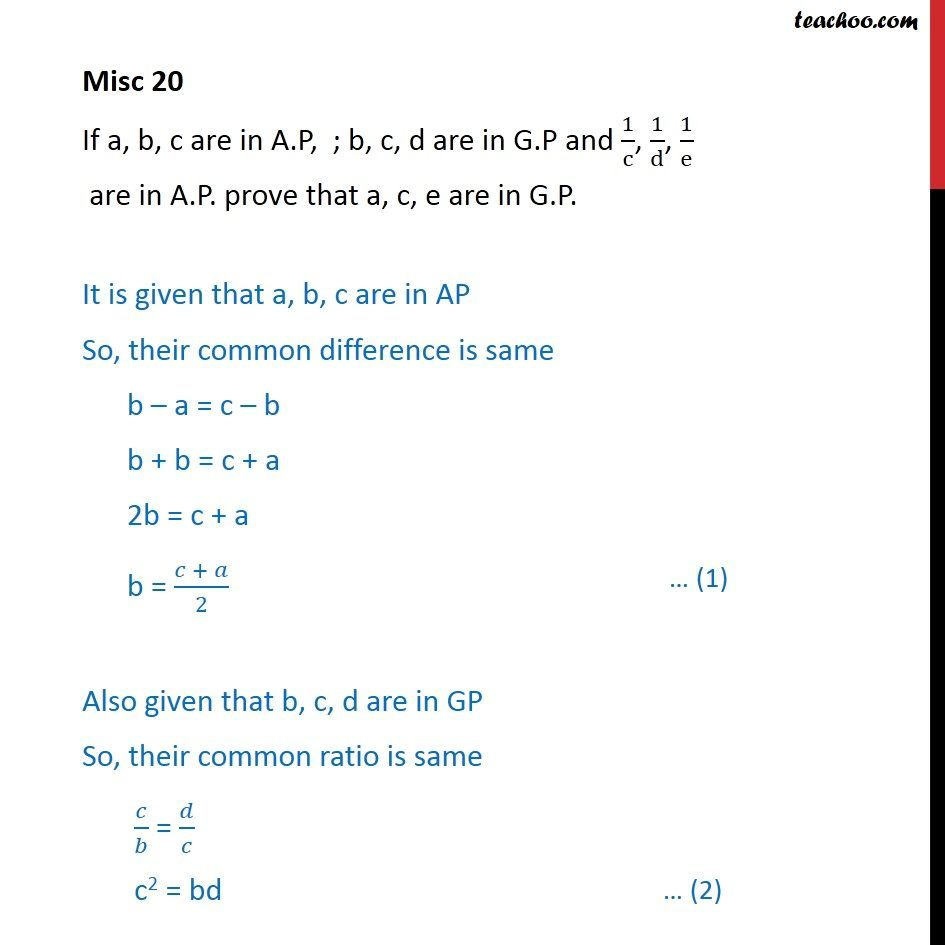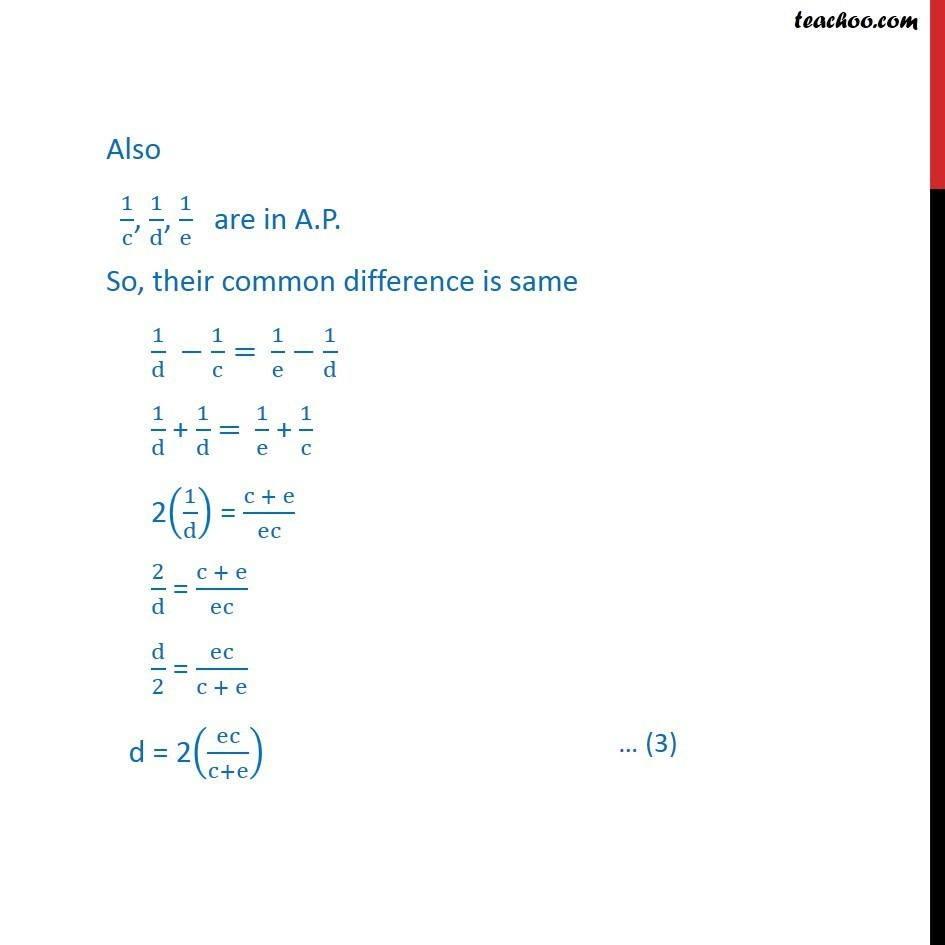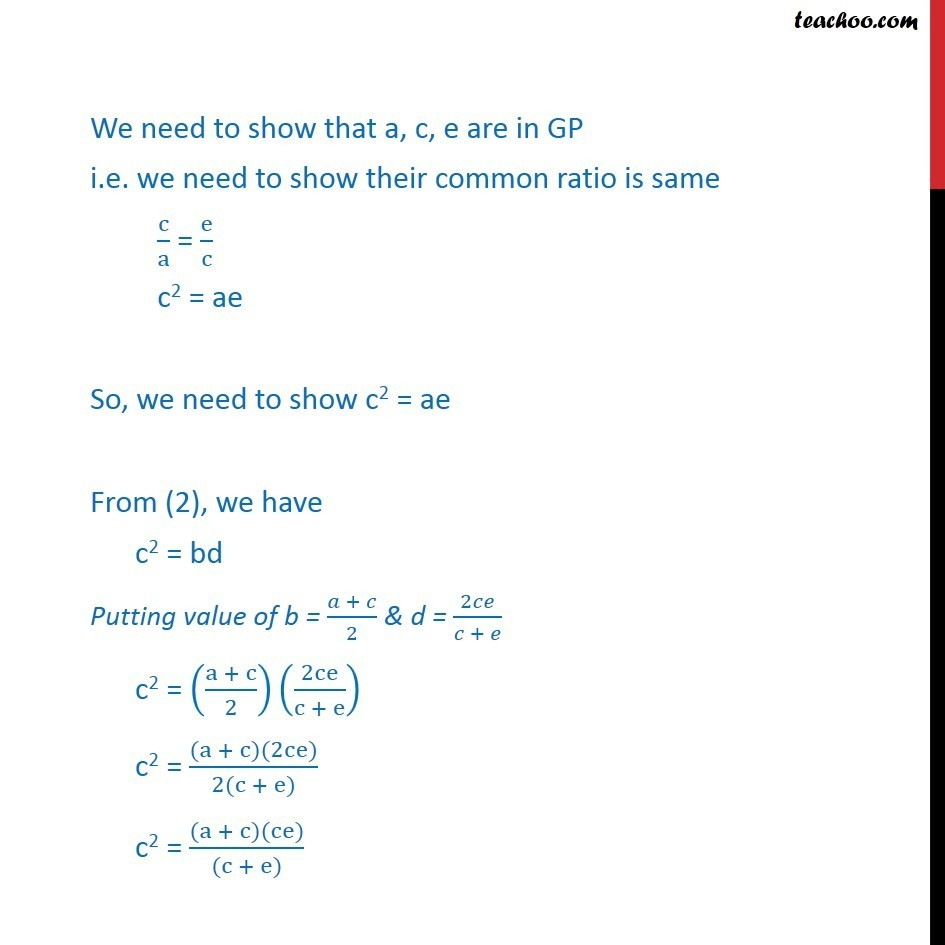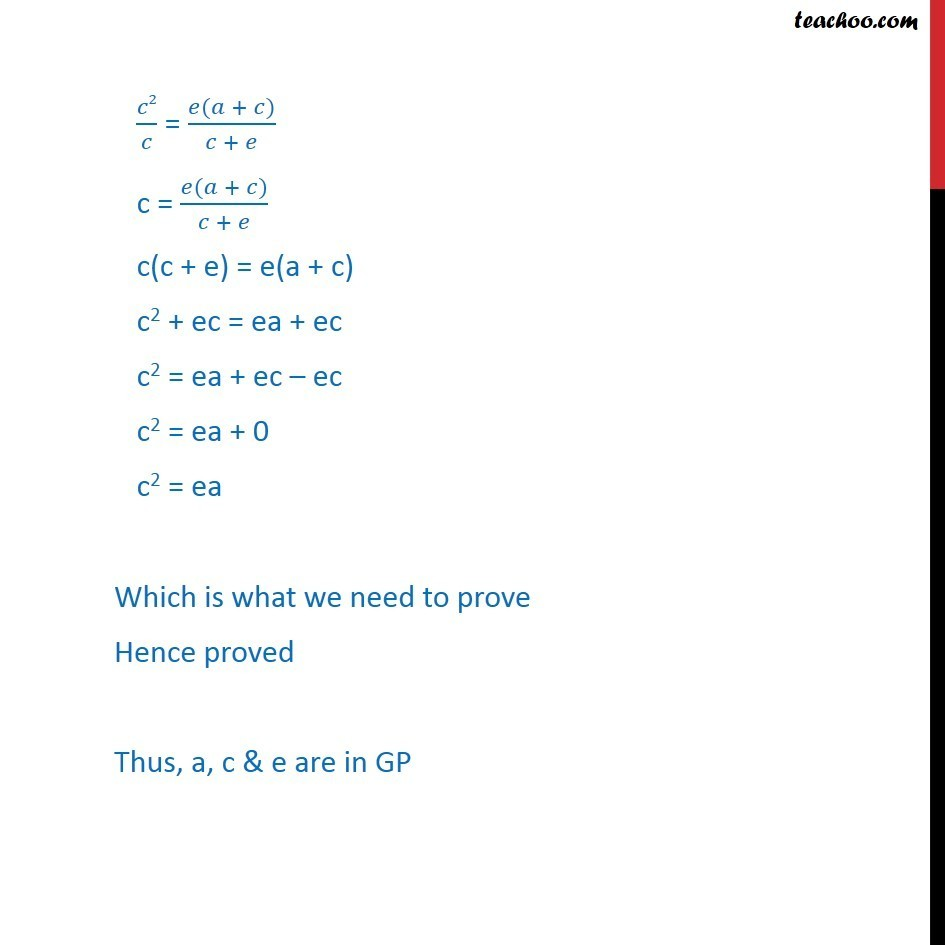Miscellaneous

Chapter 8 Class 11 Sequences and Series
Serial order wiseLearn in your speed, with individual attention - Teachoo Maths 1-on-1 Class

### Transcript

Question 10 If a, b, c are in A.P, ; b, c, d are in G.P and 1/c, 1/d, 1/e are in A.P. prove that a, c, e are in G.P. It is given that a, b, c are in AP So, their common difference is same b a = c b b + b = c + a 2b = c + a b = ( + )/2 Also given that b, c, d are in GP So, their common ratio is same / = / c2 = bd Also 1/c, 1/d, 1/e are in A.P. So, their common difference is same 1/d 1/c= 1/e 1/d 1/d + 1/d = 1/e + 1/c 2(1/d) = (c + e)/ec 2/d = (c + e)/ec d/2 = ec/(c + e) d = 2(ec/(c+e)) We need to show that a, c, e are in GP i.e. we need to show their common ratio is same c/a = e/c c2 = ae So, we need to show c2 = ae From (2), we have c2 = bd Putting value of b = ( + )/2 & d = 2 /( + ) c2 = ((a + c)/2)(2ce/(c + e)) c2 = ((a + c)(2ce))/(2(c + e)) c2 = ((a + c)(ce))/((c + e)) 2/ = ( ( + ))/( + ) c = ( ( + ))/( + ) c(c + e) = e(a + c) c2 + ec = ea + ec c2 = ea + ec ec c2 = ea + 0 c2 = ea Which is what we need to prove Hence proved Thus, a, c & e are in GP